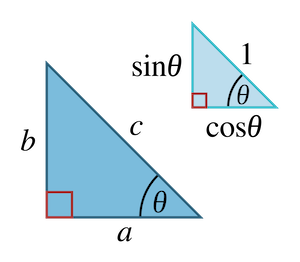## The Pythagorean triganometric identity

In this right triangle …Pythagoras’ Theorem states that $a^2 + b^2 = c^2$. Dividing both sides by $c^2$ then gives us $\frac{a^2}{c^2} + \frac{b^2}{c^2} = 1$. Since the definitions of $\sin\theta$ and $\cos\theta$ are, respectively, $\frac{b}{c}$ and $\frac{a}{c}$, the above identity follows immediately.

Simple manipulation gives us the following additional identities …

## Double angle sum identities

These follow immediately from the angle sum identities.